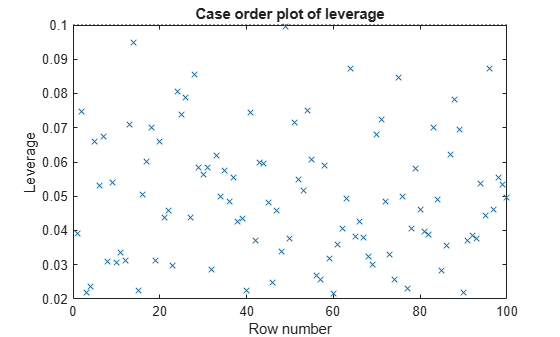Documentation

## Hat Matrix and Leverage

### Hat Matrix

#### Purpose

The hat matrix provides a measure of leverage. It is useful for investigating whether one or more observations are outlying with regard to their X values, and therefore might be excessively influencing the regression results.

#### Definition

The hat matrix is also known as the projection matrix because it projects the vector of observations, y, onto the vector of predictions, $\stackrel{^}{y}$, thus putting the "hat" on y. The hat matrix H is defined in terms of the data matrix X:

H = X(XTX)–1XT

and determines the fitted or predicted values since

`$\stackrel{^}{y}=Hy=Xb.$`

The diagonal elements of H, hii, are called leverages and satisfy

`$\begin{array}{l}0\le {h}_{ii}\le 1\\ \sum _{i=1}^{n}{h}_{ii}=p,\end{array}$`

where p is the number of coefficients, and n is the number of observations (rows of X) in the regression model. `HatMatrix` is an n-by-n matrix in the `Diagnostics` table.

#### How To

After obtaining a fitted model, say, `mdl`, using `fitlm` or `stepwiselm`, you can:

• Display the `HatMatrix` by indexing into the property using dot notation

`mdl.Diagnostics.HatMatrix`
When n is large, `HatMatrix` might be computationally expensive. In those cases, you can obtain the diagonal values directly, using

`mdl.Diagnostics.Leverage`

### Leverage

#### Purpose

Leverage is a measure of the effect of a particular observation on the regression predictions due to the position of that observation in the space of the inputs. In general, the farther a point is from the center of the input space, the more leverage it has. Because the sum of the leverage values is p, an observation i can be considered as an outlier if its leverage substantially exceeds the mean leverage value, p/n, for example, a value larger than 2*p/n.

#### Definition

The leverage of observation i is the value of the ith diagonal term, hii, of the hat matrix, H, where

H = X(XTX)–1XT.

The diagonal terms satisfy

`$\begin{array}{l}0\le {h}_{ii}\le 1\\ \sum _{i=1}^{n}{h}_{ii}=p,\end{array}$`

where p is the number of coefficients in the regression model, and n is the number of observations. The minimum value of hii is 1/n for a model with a constant term. If the fitted model goes through the origin, then the minimum leverage value is 0 for an observation at x = 0.

It is possible to express the fitted values, $\stackrel{^}{y}$, by the observed values, y, since

`$\stackrel{^}{y}=Hy=Xb.$`

Hence, hii expresses how much the observation yi has impact on ${\stackrel{^}{y}}_{i}$. A large value of hii indicates that the ith case is distant from the center of all X values for all n cases and has more leverage. `Leverage` is an n-by-1 column vector in the `Diagnostics` table.

#### How To

After obtaining a fitted model, say, `mdl`, using `fitlm` or `stepwiselm`, you can:

• Display the `Leverage` vector by indexing into the property using dot notation

`mdl.Diagnostics.Leverage`

• Plot the leverage for the values fitted by your model using

`plotDiagnostics(mdl)`
See the `plotDiagnostics` method of the `LinearModel` class for details.

### Determine High Leverage Observations

This example shows how to compute `Leverage` values and assess high leverage observations. Load the sample data and define the response and independent variables.

```load hospital y = hospital.BloodPressure(:,1); X = double(hospital(:,2:5));```

Fit a linear regression model.

`mdl = fitlm(X,y);`

Plot the leverage values.

`plotDiagnostics(mdl)`For this example, the recommended threshold value is 2*5/100 = 0.1. There is no indication of high leverage observations.